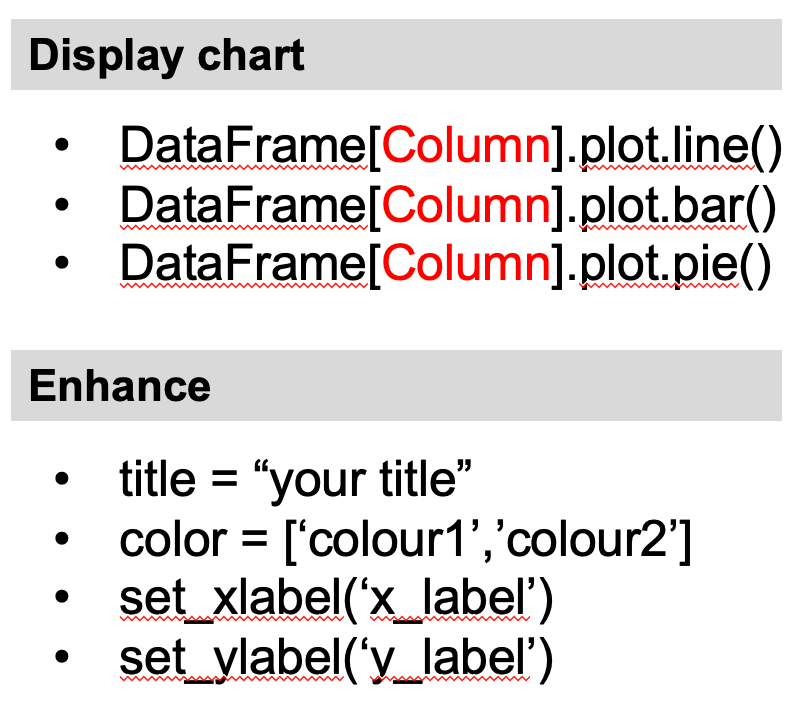# Data Analytics - Visualization

## Data Visualization

• Using charts to visualize data helps with improving understanding of a data set, interpretation of the data and communications among people who work with it.
• Pandas offers many charts that are suitable for use within management or corporate presentations.
• A chart can create a clearer picture of a set of data values than a DataFrame with rows and columns, allowing managers to incorporate this understanding into analysis and future planning.
• It is important that x-axis will be the index of your DataFrame. If you want to have a specific column to be your x-axis, then you need to set the index to that column.

## Comparison of different charts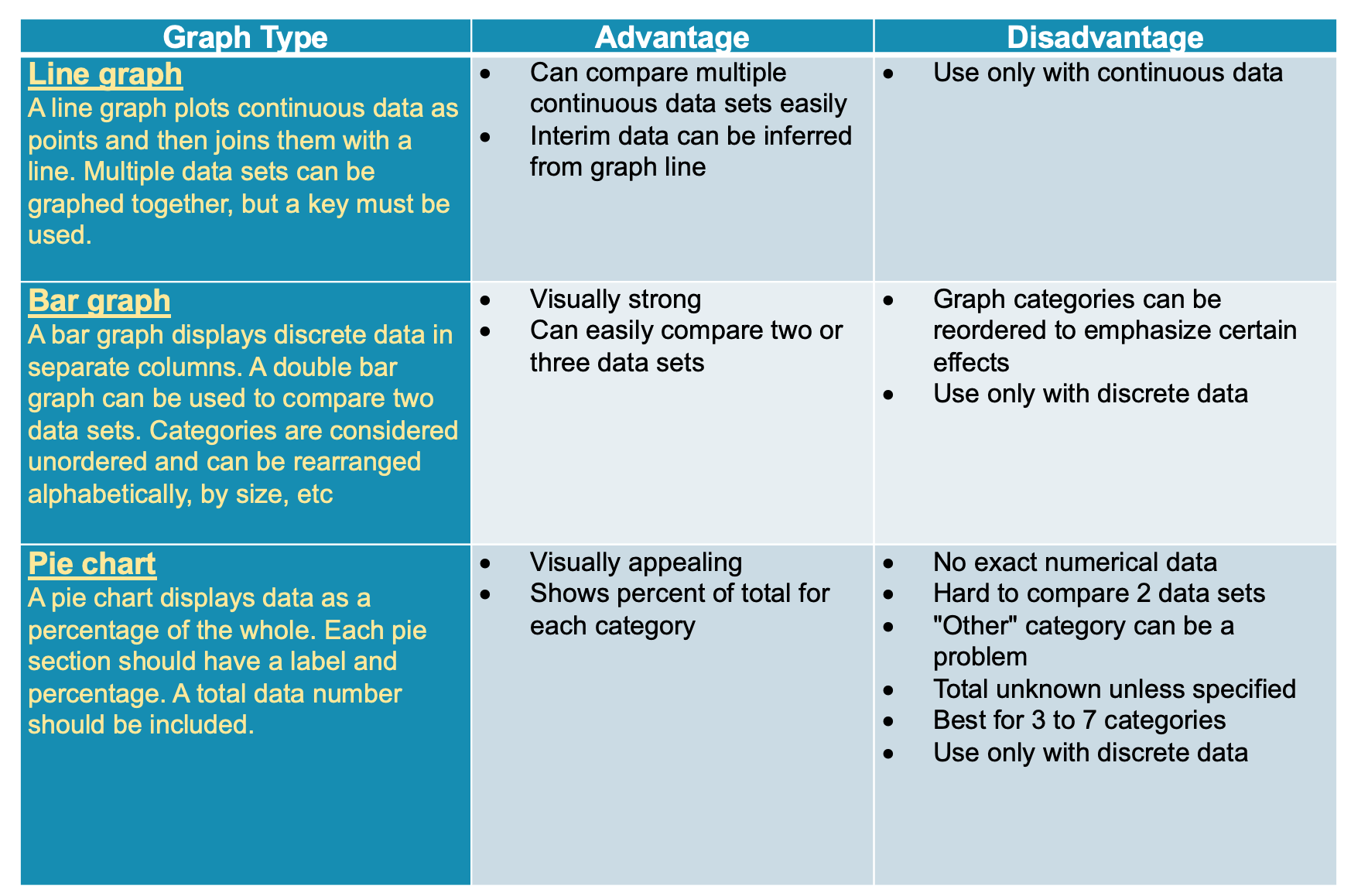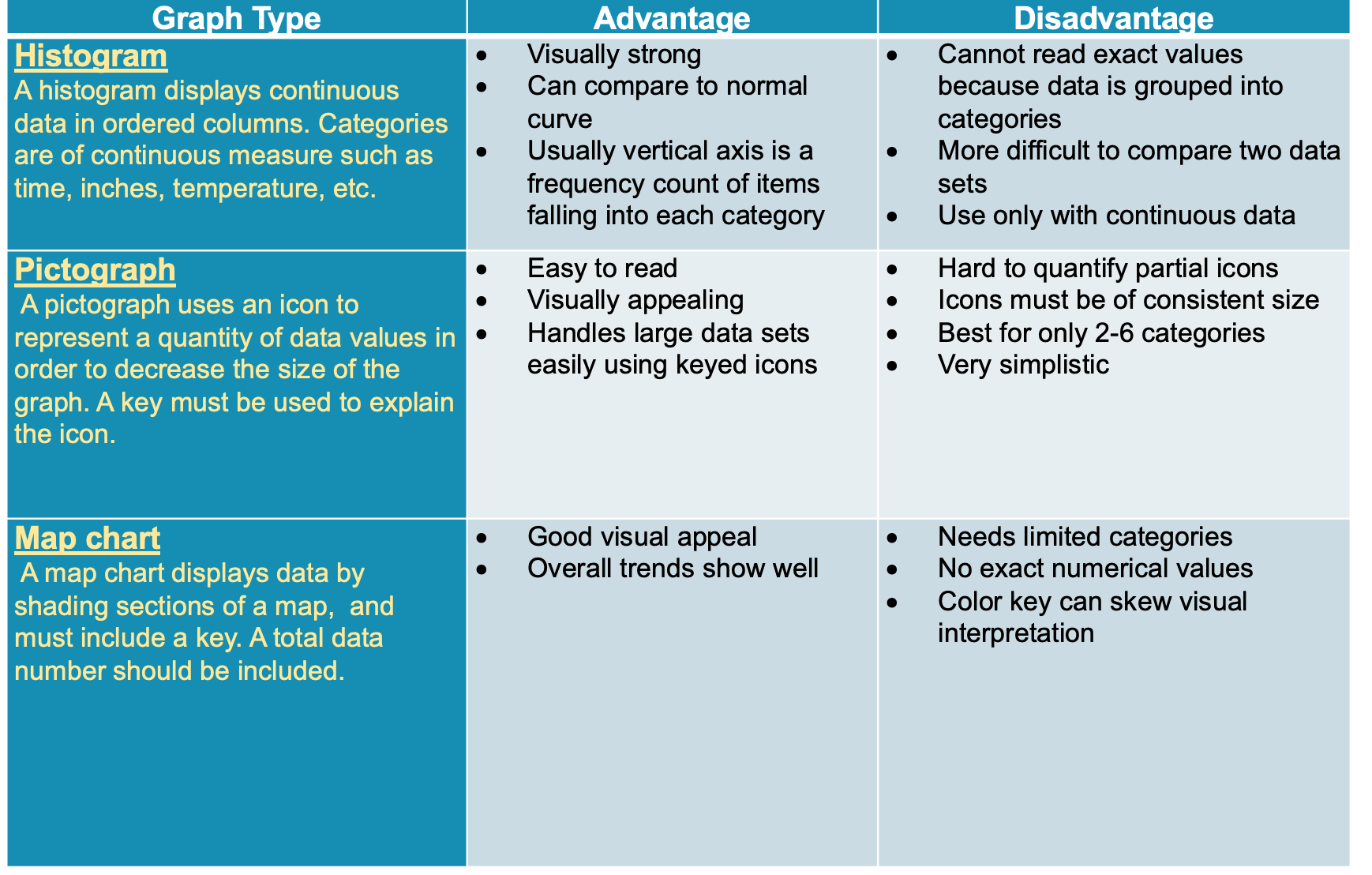## Plotting a Line Chart

A line graph plots continuous data as points and then joins them with a line. Multiple data sets can be graphed together, but a key must be used.

The structure of the command line is:
DataFrame[Column].plot()
DataFrame[Column].plot.line()

### Example: A line chart to display number of students enrolled in Undergraduate and Post Graduate

```	    df[['Graduate_UnderGraduate','Graduated_PostGraduate']].plot()
```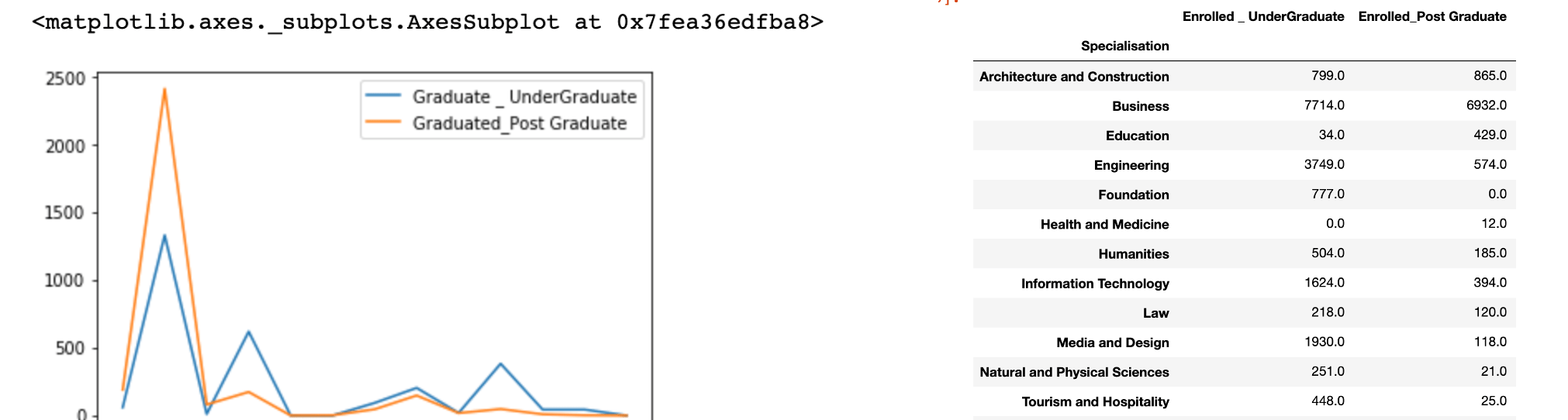## Plotting a Bar Chart

A bar graph displays discrete data in separate columns. A double bar graph can be used to compare two data sets.

The structure of the command line is:
DataFrame[Column].plot.bar()

### Example: A bar chart to display number of students enrolled in Undergraduate and Post Graduate in each Specialisation

```	    df[['Graduate _ UnderGraduate','Graduated_Post Graduate']].plot.bar()
```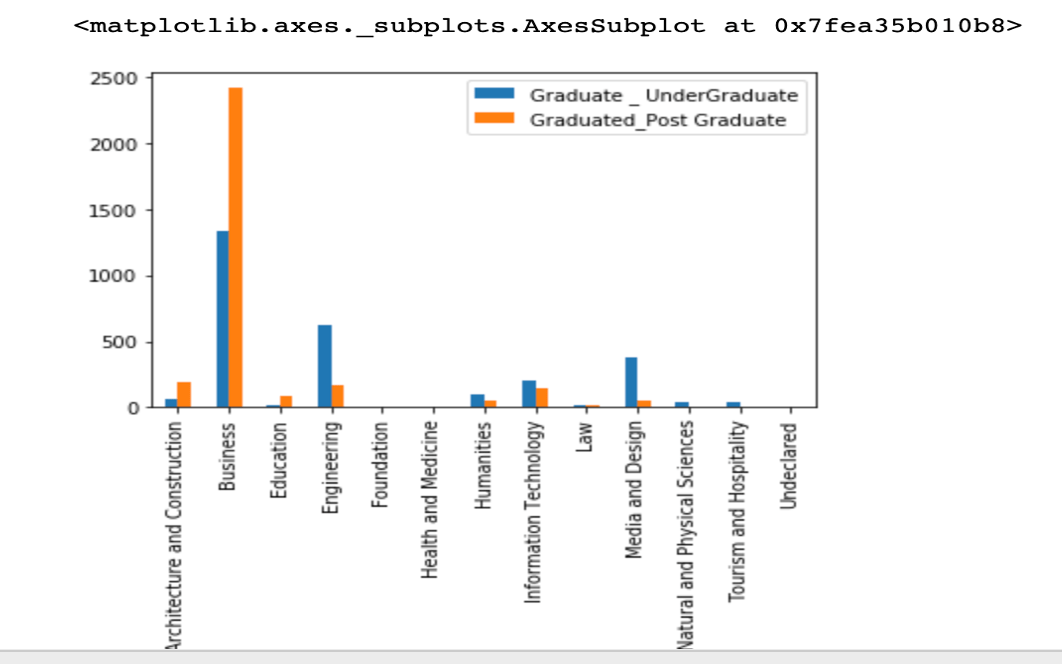## Plotting a Pie Chart

A pie chart displays data as a percentage of the whole. Each pie section should have a label and percentage. A total data number should be included.

The structure of the command line is:
DataFrame[Column].plot.pie()

### Example: A pie chart to display total number of students enrolled in each specialisation

```	    df['Total Enrolled'].plot.pie()
```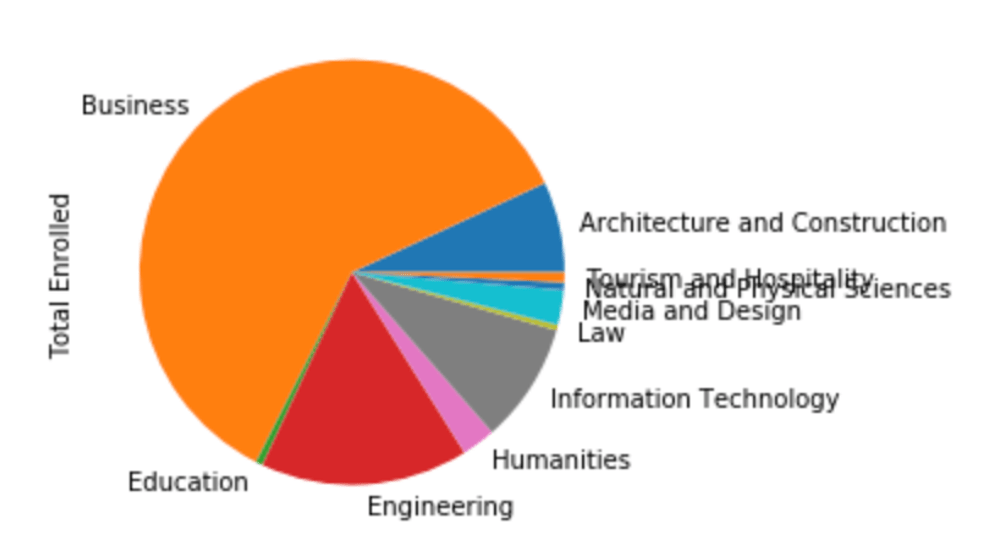## Enhancing the Chart - Example

Create a bar chart with title and change colours
```	     chart = df[['Graduate _ UnderGraduate','Graduated_Post Graduate']].plot.bar(title='Enrolled Students',color=["Blue","Green"])
```
Change the x and y label
• chart.set_xlabel("Specialisation")
• chart.set_ylabel("Enrolled")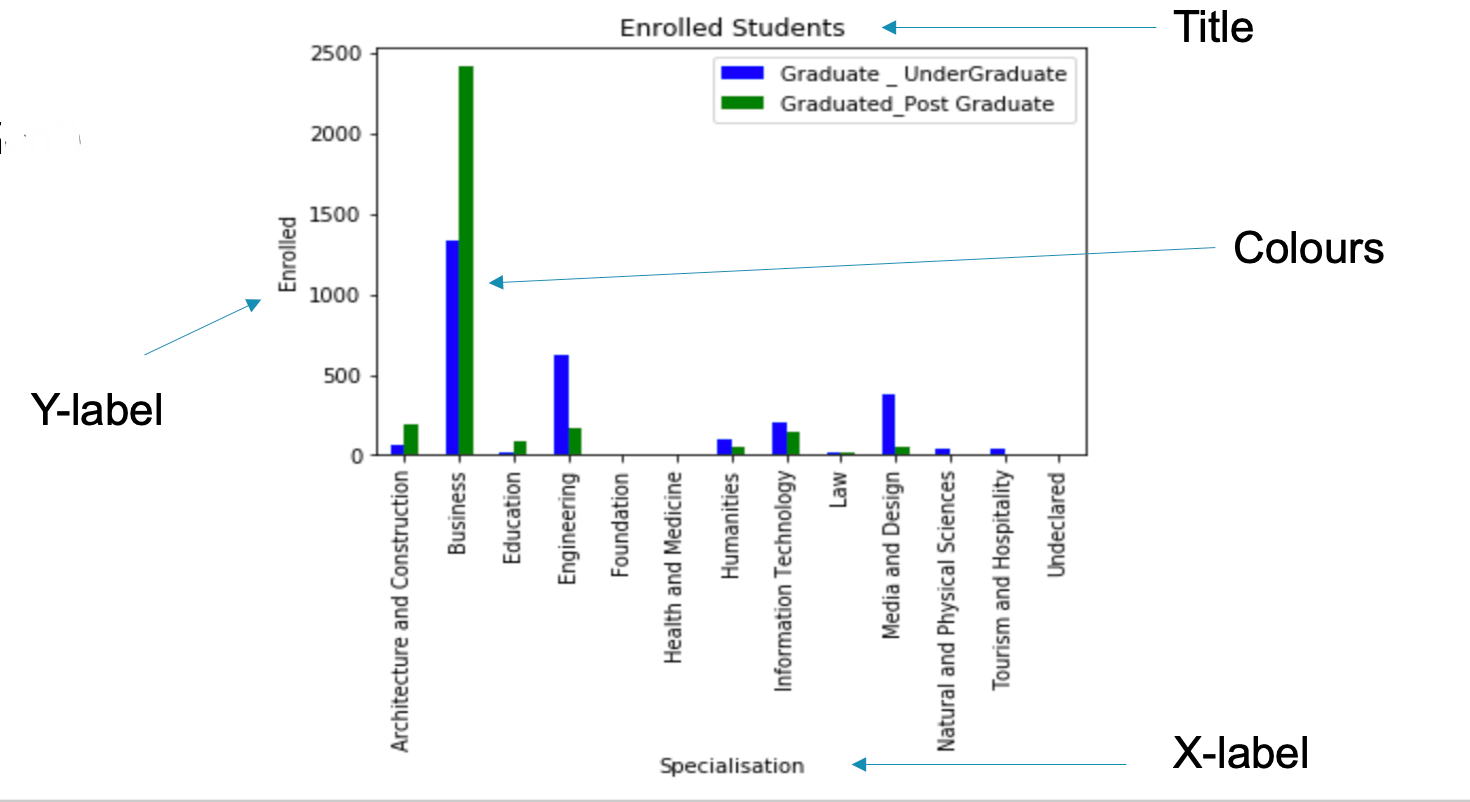## Summary of Pandas Commands